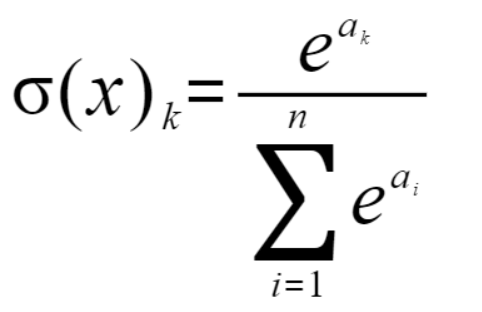# 【深度学习笔记】神经网络## 激活函数### Sigmoid函数

sigmoid函数是神经网络中最常使用的一个激活函数，它的数学表达式如下：#### sigmoid实现（基于numpy）

def sigmoid(x):
return 1/(1+np.exp(-x))

### ReLu函数

ReLu函数也是一个常用的激活函数，其特点是输出大于零时，直接输出该值，小于等于零时，输出0，其数学表达为：#### ReLu实现（基于numpy）

def relu(x):
return np.maximum(0, x)

## 神经网络的内积X = np.array([2, 3])
W = np.array([[1, 3, 5], [2, 4, 6]])

Y = np.dot(X, W)
print(Y)

[ 8 18 28]

### 例子：三层神经网络的实现

# 恒等函数
def identity_function(x):
return x#### 具体实现

# 网络的一些参数（权重和偏置）
def init_network():
network = {}
network['W1'] = np.array([[0.1, 0.2, 0.3], [0.4, 0.5, 0.6]])
network['B1'] = np.array([0.1, 0.2, 0.5])

network['W2'] = np.array([[0.1, 0.3], [0.2, 0.5], [0.4, 0.6]])
network['B2'] = np.array([[0.2, 0.7]])

network['W3'] = np.array([[0.3, 0.5], [0.4, 0.3]])
network['B3'] = np.array([0.7, 0.9])

return network

# 向前传播
def forward(network, x):
W1, W2, W3 = network['W1'], network['W2'], network['W3']
B1, B2, B3 = network['B1'], network['B2'], network['B3']

A1 = np.dot(x, W1) + B1
Z1 = sigmoid(A1)

A2 = np.dot(Z1, W2) + B2
Z2 = sigmoid(A2)

A3 = np.dot(Z2, W3) + B3
Y = identity_function(A3)

return Y

# 初始化网络
network = init_network()
# 模拟输入数据
X = np.array([0.1, 0.2])
forward(network, X)
##### 关于 forward 函数

forward原意为“向前”，这里要表示的是神经网络的“前向传播”，即信号从输入层传到输出层，与之对应的是”反向传播“，反向传播在计算梯度进行参数更新时有重要作用。

## 输出层的设计

### softmax函数

softmax函数的数学公式如下：#### 具体实现

sigmoid函数中存在指数函数，其图像如下，所以很容易导致溢出。def softmax(a):
c = np.max(a)
exp_a = np.exp(a - c)
exp_a_sum = np.sum(exp_a)
y = exp_a / exp_a_sum

return y

#### softmax函数的特性

softmax函数的输出值为0~1之间的实数，其输出总和为1（或者及其接近1），因此，通过使用softmax函数，我们可以通过概率的方式处理问题。即便是使用了softmax函数，各元素直接按的大小关系也是不变的（原来最大，现在还是最大），因为指数函数时单调递增函数，函数值的大小与a的大小成正比。一般而言，神经网络只把最大值对应的类目输出，作为识别的结果。因此，我们在有些时候可以省略输出层的函数，因为函数的计算需要额外的时间开销。